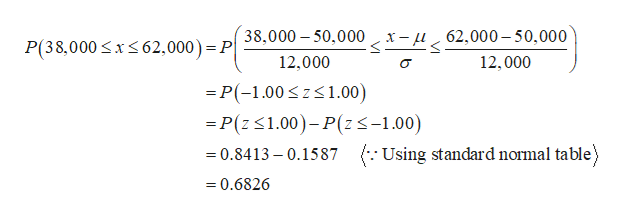# Toby's Trucking Company determined that on an annual basis, the distance traveled per truck is normally distributed, with a mean of 50,000 miles and a standard deviation of 12,000 miles.What proportion of trucks can be expected to travel between 38,000 and 62,000 miles in the year?What percentage of the trucks travel less than 35,000 miles in the year?What percentage of the trucks travel more than 57,000 miles in the year?How many miles will be traveled by at least (equal to and more than) 72% of the trucks?Your Answers:_____________ B. _____________    C.   _____________      D. ________  Warranty records show that the probability that a new car needs a warranty repair in the first 90 days is 0.05.  If a sample of 3 new cars is selected:What is the probability that none needs a warranty repair? __________________What is the probability that at least one needs a warranty repair? _________________What is the probability that more than one needs a warranty repair? __________________The key components of this problem are:Sample size (trials) = 3 new carsProbability of “success” = 5%Number of “successes”:A: Exactly 0B: 1 or moreC: More than one

Question
1. Toby's Trucking Company determined that on an annual basis, the distance traveled per truck is normally distributed, with a mean of 50,000 miles and a standard deviation of 12,000 miles.
1. What proportion of trucks can be expected to travel between 38,000 and 62,000 miles in the year?
2. What percentage of the trucks travel less than 35,000 miles in the year?
3. What percentage of the trucks travel more than 57,000 miles in the year?
4. How many miles will be traveled by at least (equal to and more than) 72% of the trucks?

1. _____________ B. _____________    C.   _____________      D. ________

1. Warranty records show that the probability that a new car needs a warranty repair in the first 90 days is 0.05.  If a sample of 3 new cars is selected:
1. What is the probability that none needs a warranty repair? __________________
2. What is the probability that at least one needs a warranty repair? _________________
3. What is the probability that more than one needs a warranty repair? __________________

The key components of this problem are:
Sample size (trials) = 3 new cars
Probability of “success” = 5%
Number of “successes”:

A: Exactly 0
B: 1 or more
C: More than one

check_circle

Step 1

Hey there! Thank you for posting the question. Since there are multiple questions posted, we will answer first question. If you want any specific question to be answered then please submit that question only or specify the question number in your message.

It is given that the mean and standard deviation are 50,000 and 12,000, respectively.

Step 2

1)

The required proportion is obtained as follows:help_outlineImage Transcriptionclose38,000 50,000 x-u62,000-50,000 P(38,000 xs62,000) = P 12,000 12,000 -P(-1.00zs1.00) =P(z1.00)-P(zs-1.00) =0.8413 - 0.1587 Using standard normal table) 0.6826 fullscreen
Step 3

2)

The required percentage is o...

### Want to see the full answer?

See Solution

#### Want to see this answer and more?

Solutions are written by subject experts who are available 24/7. Questions are typically answered within 1 hour.*

See Solution
*Response times may vary by subject and question.
Tagged in

### Statistics